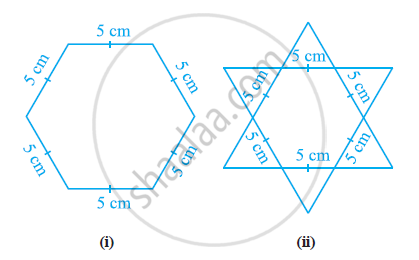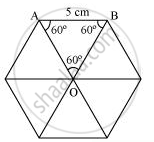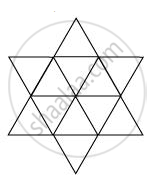Complete the hexagonal and star shaped rangolies (see the given figures) by filling them with as many equilateral triangles of side 1 cm as you can. Count the number of triangles in each case. Which has more triangles? - Mathematics

Complete the hexagonal and star shaped rangolies (see the given figures) by filling them with as many equilateral triangles of side 1 cm as you can. Count the number of triangles in each case. Which has more triangles?Solution

It can be observed that hexagonal-shaped rangoli has 6 equilateral triangles in it."Area of "ΔOAB = sqrt3/4"(side)"^2

= sqrt3/4(5)^2

= sqrt3/4(25)

= (25sqrt3)/4cm^2

"Area of hexagonal-shaped rangoli "=6xx(25sqrt3)/4=(75sqrt3)/2cm^2

"Area of equilateral triangle having its side as 1cm "=sqrt3/4(1)^2=sqrt3/4cm^2

"Number of equilateral triangles of 1 cm side that can be filled in this hexagonal-shaped rangoli "=((75sqrt3)/2)/(sqrt3/4)=150

Star-shaped rangoli has 12 equilateral triangles of side 5 cm in it."Area of star-shaped rangoli "=12xxsqrt3/4xx(5)^2=75sqrt3

"Number of equilateral triangles of 1 cm side that can be filled in this star-shaped rangoli "=(75sqrt3)/(sqrt3/4)=300

Therefore, star-shaped rangoli has more equilateral triangles in it.

Concept: Inequalities in a Triangle
Is there an error in this question or solution?

APPEARS IN

NCERT Class 9 Maths
Chapter 7 Triangles
Exercise 7.5 | Q 4 | Page 133